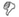mathematics love

# mathematics

## Definitions

### from The American Heritage® Dictionary of the English Language, 5th Edition.

• noun The study of the measurement, properties, and relationships of quantities and sets, using numbers and symbols.

### from The Century Dictionary.

• noun The science of quantity; the study of ideal constructions (often applicable to real problems), and the discovery thereby of relations between the parts of these constructions, before unknown.

### from the GNU version of the Collaborative International Dictionary of English.

• noun That science, or class of sciences, which treats of the exact relations existing between quantities or magnitudes, and of the methods by which, in accordance with these relations, quantities sought are deducible from other quantities known or supposed; the science of spatial and quantitative relations.

• noun An abstract representational system used in the study of numbers, shapes, structure and change and the relationships between these concepts.
• noun A person's ability to count, calculate, and use different systems of mathematics at differing levels.

• noun a science (or group of related sciences) dealing with the logic of quantity and shape and arrangement

## Etymologies

### from The American Heritage® Dictionary of the English Language, 4th Edition

[From Middle English mathematik, from Old French mathematique, from Latin mathēmatica, from Greek mathēmatikē (tekhnē), mathematical (science), feminine of mathēmatikos, mathematical; see mathematical.]

From Latin mathēmatica ("mathematics"), from Ancient Greek μαθηματικός (mathematikos, "fond of learning"), from μάθημα (máthema, "knowledge, study, learning").

## Support## Examples

• A reflection of their fundamental philosophy, the Pythagoreans invented the term mathematics, from the Greek word mathema, which meant “science.”

Euclid’s Window Leonard Mlodinow 2001

• A reflection of their fundamental philosophy, the Pythagoreans invented the term mathematics, from the Greek word mathema, which meant “science.”

Euclid’s Window Leonard Mlodinow 2001

• A reflection of their fundamental philosophy, the Pythagoreans invented the term mathematics, from the Greek word mathema, which meant “science.”

Euclid’s Window Leonard Mlodinow 2001

• Considered a child prodigy, he went to Harvard and graduated, then he got his PhD in mathematics from the University of Michigan.

• Education: Bachelor's degree in mathematics from the University of Delhi and an MBA in marketing and finance from the Indian Institute of Management-Ahmedabad.

• Only a philosophical topology, analogous to what in mathematics is defined as analysis situ (analysis of site), in opposition to analysis magnitudinis

• Regarding an underlying mathematical edifice, a possible analogy in mathematics is the existence of non-computable numbers, these numbers have no deterministic, no algorithmic description, yet they exist.

• Regarding an underlying mathematical edifice, a possible analogy in mathematics is the existence of non-computable numbers, these numbers have no deterministic, no algorithmic description, yet they exist.

• The question asked which branch of mathematics comes from the Greek word for reunite.

• We compose our systems of music, which we call mathematics, that are model systems of internal consistency.

Do Atheists Exist? Sean 2008

• 9 × 9 + 7 = 88

98 × 9 + 6 = 888

987 × 9 + 5 = 8888

9876 × 9 + 4 = 88888

98765 × 9 + 3 = 888888

987654 × 9 + 2 = 8888888

9876543 × 9 + 1 = 88888888

98765432 × 9 + 0 = 888888888

987654321 x 9 - 1 = 8888888888

--From: Futility Closet

(BTW, 80/81 = .987654321 with rounding)

July 15, 2008• "Mathematics, rightly viewed, possesses not only truth, but supreme beauty — a beauty cold and austere, like that of sculpture, without appeal to any part of our weaker nature, without the gorgeous trappings of painting or music, yet sublimely pure, and capable of a stern perfection such as only the greatest art can show. The true spirit of delight, the exaltation, the sense of being more than Man, which is the touchstone of the highest excellence, is to be found in mathematics as surely as poetry."

-Bertrand Russell

Yes. Yes indeed.

April 24, 2010• A person steps into the road, turns, sees a truck about to hit him, seems to freeze. The freezing is the amazingly quick brain making its honourable start on the avoidance mathematics, the geometry of getting out of the way. And even the amazingly quick brain is too slow. The first trajectory calculations are barely - BAM! Goodnight. From "The Last Werewolf" by Glen Duncan.

March 23, 2012